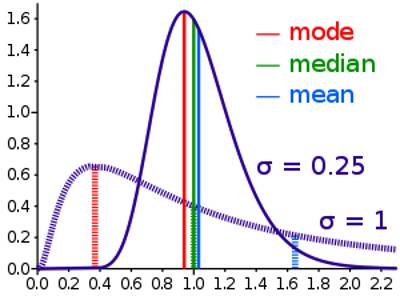Statistical Median

The median is a simple measure of central tendency. To find the median, we arrange the observations in order from smallest to largest value. If there is an odd number of observations, the median is the middle value. If there is an even number of observations, the median is the average of the two middle values.

The statistical median is the middle number of a group of numbers that have been arranged in order by size. If there is an even number of terms, the median is the mean of the two middle numbers

To find the median of a group of numbers:

• Arrange the numbers in order by size
• If there is an odd number of terms, the median is the center term.
• If there is an even number of terms, add the two middle terms and divide by 2.

In the data center, means and medians are often tracked over time to spot trends, which inform capacity planning or power cost predictions.The statistical median is the middle number in a sequence of numbers. To find the median, organize each number in order by size; the number in the middle is the median. For the five servers in the rack, arrange the power consumption figures from lowest to highest: 90 W, 98 W, 100 W, 102 W and 105 W. The median power consumption of the rack is 100 W. If there is an even set of numbers, average the two middle numbers. For example, if the rack had a sixth server that used 110 W, the new number set would be 90 W, 98 W, 100 W, 102 W, 105 W and 110 W. Find the median by averaging the two middle numbers: (100 + 102)/2 = 101 W.Calculating the median

If observations of a variable are ordered by value, the median value corresponds to the middle observation in that ordered list. The median value corresponds to a cumulative percentage of 50% (i.e., 50% of the values are below the median and 50% of the values are above the median). The position of the median is

{(n + 1) ÷ 2}th value, where n is the number of values in a set of data.

In order to calculate the median, the data must first be ranked (sorted in ascending order). The median is the number in the middle.

Median = the middle value of a set of ordered data.

The median is usually calculated for numeric variables, but may also be calculated for categorical variables that are sequenced, such as the categories in a satisfaction survey: excellent, good, satisfactory and poor. These qualitative categories can be ranked in order, and thus, are considered ordinal.

Example – Raw data (discrete variables)

Now, if the runner sprints the sixth 200-meter race in 24.7 seconds, what is the median value now?

Again, you first put the data in ascending order: 24.7, 25.0, 25.2, 25.6, 25.7, 26.1. Then, you use the same formula to calculate the median time.

Median = {(n + 1) ÷ 2}th value

= (6 + 1) ÷ 2

= 7 ÷ 2

= 3,5

Since there is an even number of observations in this data set, there is no longer a distinct middle value. The median is the 3.5th value in the data set meaning that it lies between the third and fourth values. Thus, the median is calculated by averaging the two middle values of 25.2 and 25.6. Use the formula below to get the average value.

Average = (value below median + value above median) ÷ 2

= (third value + fourth value) ÷ 2

= (25.2 + 25.6) ÷ 2

= 50.8 ÷ 2

= 25.4

The value 25.4 falls directly between the third and fourth values in this data set, so 25.4 seconds would be the median time.

Information Source: